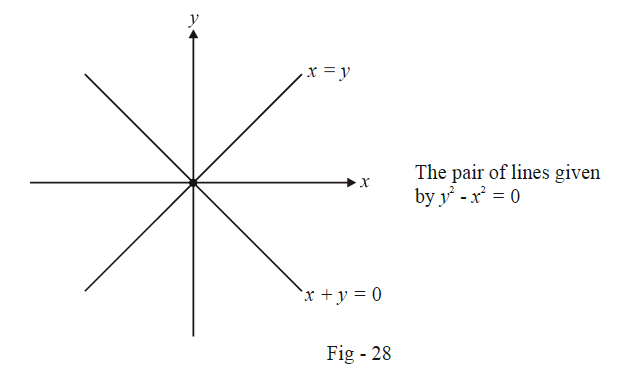# Introduction to Pairs of Lines

Go back to  'Straight Lines'

Consider two lines

\begin{align} {L_1} \equiv y - {m_1}x - {c_1} = 0\\ {L_2} \equiv y - {m_2}x - {c_2} = 0 \end{align}

What do you think will $${L_1}{L_2} = 0$$ represent? It is obvious that any point lying on $${L_1}$$ and $${L_2}$$ will satisfy $${L_1}{L_2} = 0,$$ and thus $${L_1}{L_2} = 0$$ represents the set of points constituting both the lines, i.e.,

$\boxed{{L_1}{L_2} = 0{\text{ represents the pair of straight lines given by }}{L_1} = 0\,\,{\text{and }}{L_2} = 0}$

For example, consider the equation $${y^2} - {x^2} = 0.$$ What does this represent ? We have

\begin{align} \qquad & {y^2} - {x^2} = 0 \qquad \qquad \qquad \qquad...(1)\\ \Rightarrow \qquad & (y + x)(y - x) = 0\\ \Rightarrow \qquad & (1)\;{\rm{represents}}\;{\text{the pair of straight lines}}\;x = y\;{\rm{and}}\;x + y = 0. \end{align}There is nothing special about considering a pair. We can similarly define the joint equation of $$n$$ straight lines $${L_i} \equiv y - {m_i}x - {c_i} = 0\;(i = 1,\,2...,n)$$ as

\begin{align} & {L_1}{L_2}...{L_n} = 0\\ \Rightarrow \qquad & (y - {m_1}x - {c_1})(y - {m_2}x - {c_2})...(y - {m_n}x - {c_n}) = 0 \qquad \qquad \qquad ...(2) \end{align}

Any point lying on any of these n straight lines will satisfy (2), and thus (2) represents the set of all points constituting the n lines, i.e. (2) represents the joint equation of the n straight lines.

What is relevant to us at this stage is only a pair of straight lines and it is on a pair of lines that we now focus our attention.